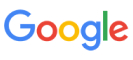New update is available. Click here to update.
Topics

# Search Pattern (Rabin-Karp Algorithm)

Moderate0/80
Average time to solve is 20m## Problem statement

You’re given two strings, 'text' of length 'n' and 'pattern' of length 'm', consisting of lowercase characters.

Find all the occurrences of the string ‘pattern’ in ‘text’.

For each occurrence, print the index from where it starts in the string ‘text’ (1 - indexed).

Example:
``````Input: ‘text’ = “cxyzghxyzvjkxyz” and ‘pattern’ = “xyz”

Output: 2 7 13

Explanation: The pattern ‘pattern’ = “xyz” appears at 3 positions in ‘text’.
``````
Detailed explanation ( Input/output format, Notes, Images )
Sample Input 1:
``````cxyzghxyzvjkxyz
xyz
``````

Sample Output 1:
``````3
2 7 13
``````

Explanation Of Sample Input 1 :
``````The pattern ‘pattern’ = “xyz” appears at 3 positions in ‘text’.
``````

Sample Input 2 :
``````ababacabab
aba
``````

Sample Output 2 :
``````3
1 3 7
``````

Explanation Of Sample Input 2 :
``````Here we have an overlap between the first occurrence (at position 1) and the second occurrence (at position 3), and we are considering both.
``````

Sample Input 3 :
``````abcd
xy
``````

Sample Output 3 :
``````0
``````

Expected time complexity:
``````The expected time complexity is O(‘n’ + ‘m’).
``````

Constraints:
``````1 <= ‘n’ <= 10^5
1 <= ‘m’ <= ‘n’
``````Console